# 拉普拉斯变换

时域函数的乘以e -st

## 拉普拉斯变换功能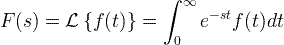## 拉普拉斯变换表

ft

Fs）= L { ft）}

Ť ñ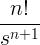Ť

Γ（一个+1）⋅小号- （+1）

Ë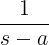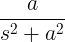cos at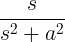SINH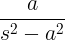COSH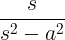Ť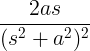t cos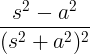Ë -atωT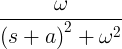Ë -at COS ωT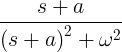δ（

1

δ（ta

Ë -as

ft

Fs

## 拉普拉斯变换的例子

#### 例子1

ft）= 3 t + 2 t 2

ℒ{ t } = 1 / s 2

ℒ{ t 2 } = 2 / s 3

Fs）=ℒ{ ft）} =ℒ{3 t + 2 t 2 } =3ℒ{ t } +2ℒ{ t 2 } = 3 / s 2 + 4 / s 3

#### 范例＃2

Fs）= 3 /（s 2 + s -6）

Fs）= 3 /（s 2 + s -6）= 3 / [（s -2）（s +3）] = a /（s -2）+ b /（s +3）

[ as +3）+ bs -2）] / [（s -2）（s +3）] = 3 / [（s -2）（s +3）]

as +3）+ bs -2）= 3

a + bs + 3 a -2 b = 3

a + b = 0，3 a -2 b = 3

a = 3/5，b = -3/5

Fs）= 3/5（s -2）-3/5 （s +3）

ft）=（3/5）e 2 t-（3/5）e -3 t